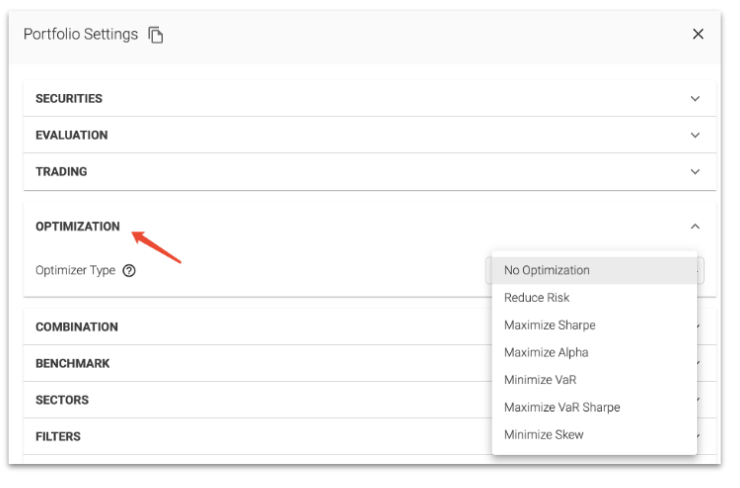# Portfolio Performance Techniques - Optimization

There are two types of Optimization: in model settings and in portfolio settings.

Optimization in model settings is used to sort your universe from best to worst performing stocks. In portfolio settings, Optimization adds a secondary goal by adjusting the weights of your portfolio holdings.

To add a secondary optimization, you start by selecting an Optimizer Type• Reduce Risk - Reduces portfolio volatility.
• Maximize Sharpe - Maximizes Sharpe using out-of-sample** estimates for expected return.
• Maximize Sharpe V2 - Maximizes exposure to the underlying signals while minimizing exposure to volatility.
• Maximize Alpha - Maximizes expected return using out-of-sample** estimates for expected return
• Maximize Alpha V2 - Maximizes exposure to underlying signal with no consideration to volatility.
• Max VaR Sharpe - Maximizes Value at Risk by looking at the proposed portfolio over the last year and maximizing Sharpe by observed return vs observed volatility.
• Max VaR Sharpe V2 - Maximizes Value at Risk by looking at the proposed portfolio over the last year and maximizing Sharpe by maximizing signal exposure vs historically observed volatility.
• Minimize Skew - Minimizes skew by finding portfolio weightings where returns are closer to the mean.
• Minimize Value at Risk - Minimizes Value at Risk by looking at the proposed portfolio over the last year and minimizing drawdowns.

**Out-of-sample estimate: estimate made by a model on data not used during the training of the model